### 5. UNDERSTANDING COSMIC ACCELERATION

Understanding the origin of cosmic acceleration presents a stunning opportunity for theorists. As discussed in Section 2, a smooth component with large negative pressure has repulsive gravity and can lead to the observed accelerated expansion within the context of GR. This serves to define dark energy. There is no shortage of ideas for what dark energy might be, from the quantum vacuum to a new, ultra-light scalar field. Alternatively, cosmic acceleration may arise from new gravitational physics, perhaps involving extra spatial dimensions. Here, we briefly review the theoretical landscape.

5.1.1. VACUUM ENERGY     Vacuum energy is simultaneously the most plausible and most puzzling dark energy candidate. General covariance requires that the stress-energy of the vacuum takes the form of a constant times the metric tensor, TVACµ=VAC gµ. Because the diagonal terms (T00, Tii) of the stress-energy tensor Tµ are the energy density and minus the pressure of the fluid, and gµ is just the Kronecker delta, the vacuum has a pressure equal to minus its energy density, pVAC = -VAC. This also means that vacuum energy is mathematically equivalent to a cosmological constant.

Attempts to compute the value of the vacuum energy density lead to very large or divergent results. For each mode of a quantum field there is a zero-point energy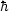/ 2, so that the energy density of the quantum vacuum is given by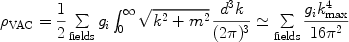(17)

where gi accounts for the degrees of freedom of the field (the sign of gi is + for bosons and - for fermions), and the sum runs over all quantum fields (quarks, leptons, gauge fields, etc). Here kmax is an imposed momentum cutoff, because the sum diverges quartically.

To illustrate the magnitude of the problem, if the energy density contributed by just one field is to be at most the critical density, then the cutoff kmax must be < 0.01 eV — well below any energy scale where one could have appealed to ignorance of physics beyond. [Pauli apparently carried out this calculation in the 1930's, using the electron mass scale for kmax and finding that the size of the Universe, that is, H-1, "could not even reach to the moon" [Straumann 2002].] Taking the cutoff to be the Planck scale (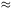1019 GeV), where one expects quantum field theory in a classical spacetime metric to break down, the zero-point energy density would exceed the critical density by some 120 orders-of-magnitude! It is very unlikely that a classical contribution to the vacuum energy density would cancel this quantum contribution to such high precision. This very large discrepancy is known as the cosmological constant problem [Weinberg 1989].

Supersymmetry, the hypothetical symmetry between bosons and fermions, appears to provide only partial help. In a supersymmetric (SUSY) world, every fermion in the standard model of particle physics has an equal-mass SUSY bosonic partner and vice versa, so that fermionic and bosonic zero-point contributions toVAC would exactly cancel. However, SUSY is not a manifest symmetry in Nature: none of the SUSY particles has yet been observed in collider experiments, so they must be substantially heavier than their standard-model partners. If SUSY is spontaneously broken at a mass scale M, one expects the imperfect cancellations to generate a finite vacuum energy densityVAC ~ M4. For the currently favored value M ~ 1 TeV, this leads to a discrepancy of 60 (as opposed to 120) orders of magnitude with observations. Nonetheless, experiments at the Large Hadron Collider (LHC) at CERN will soon begin searching for signs of SUSY, e.g., SUSY partners of the quarks and leptons, and might shed light on the vacuum-energy problem.

Another approach to the cosmological constant problem involves the idea that the vacuum energy scale is a random variable that can take on different values in different disconnected regions of the Universe. Because a value much larger than that needed to explain the observed cosmic acceleration would preclude the formation of galaxies (assuming all other cosmological parameters are held fixed), we could not find ourselves in a region with such largeVAC [Weinberg 1987]. This anthropic approach finds a possible home in the landscape version of string theory, in which the number of different vacuum states is very large and essentially all values of the cosmological constant are possible. Provided that the Universe has such a multiverse structure, this might provide an explanation for the smallness of the cosmological constant [Bousso & Polchinski 2000, Susskind 2003].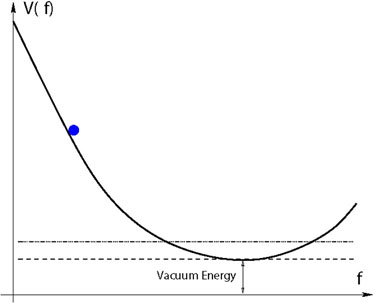Figure 10. Generic scalar potential V(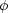). The scalar field rolls down the potential eventually settling at its minimum, which corresponds to the vacuum. The energy associated with the vacuum can be positive, negative, or zero.

5.1.2. SCALAR FIELDS     Vacuum energy does not vary with space or time and is not dynamical. However, by introducing a new degree of freedom, a scalar field, one can make vacuum energy effectively dynamical [Ratra & Peebles 1988, Wetterich 1988, Frieman et al. 1995, Zlatev, Wang & Steinhardt 1992]. For a scalar field, with Lagrangian density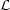= 1/2µµ- V(), the stress-energy takes the form of a perfect fluid, with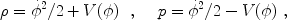(18)

whereis assumed to be spatially homogeneous, i.e.,(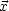, t) =(t),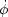2/2 is the kinetic energy, and V() is the potential energy; see Fig. 10. The evolution of the field is governed by its equation of motion,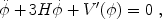(19)

where a prime denotes differentiation with respect to. Scalar-field dark energy can be described by the equation-of-state parameter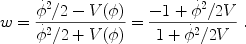(20)

If the scalar field evolves slowly,2 / 2V << 1, then w-1, and the scalar field behaves like a slowly varying vacuum energy, withVAC(t)V[(t)]. In general, from Eq. (20), w can take on any value between -1 (rolling very slowly) and +1 (evolving very rapidly) and varies with time.

Many scalar field models can be classified dynamically as "thawing" or "freezing" [Caldwell & Linder 2005]. In freezing models, the field rolls more slowly as time progresses, i.e., the slope of the potential drops more rapidly than the Hubble friction term 3Hin Eq. (19). This can happen if, e.g., V() falls off exponentially or as an inverse power-law at large. For thawing models, at early times the field is frozen by the friction term, and it acts as vacuum energy; when the expansion rate drops below H2 = V''(), the field begins to roll and w evolves away from -1. The simplest example of a thawing model is a scalar field of mass m, with V() = m22/2. Since thawing and freezing fields tend to have different trajectories of w(z), precise cosmological measurements might be able to discriminate between them.

5.1.3. COSMIC COINCIDENCE AND SCALAR FIELDS     As Fig. 1 shows, through most of the history of the Universe, dark matter or radiation dominated dark energy by many orders of magnitude. We happen to live around the time that dark energy has become important. Is this coincidence betweenDE andM an important clue to understanding cosmic acceleration or just a natural consequence of the different scalings of cosmic energy densities and the longevity of the Universe? In some freezing models, the scalar field energy density tracks that of the dominant component (radiation or matter) at early times and then dominates at late times, providing a dynamical origin for the coincidence. In thawing models, the coincidence is indeed transitory and just reflects the mass scale of the scalar field.

5.1.4. MORE COMPLICATED SCALAR-FIELD MODELS     While the choice of the potential V() allows a large range of dynamical behaviors, theorists have also considered the implications of modifying the canonical form of the kinetic energy term 1/2µµin the Lagrangian. By changing the sign of this term, from Eq. 20 it is possible to have w < -1 [Caldwell 2002], although such theories are typically unstable [Carroll, Hoffman & Trodden 2003]. In "k-essence," one introduces a field-dependent kinetic term in the Lagrangian to address the coincidence problem [Armendariz-Picon, Mukhanov & Steinhardt 2000].

5.1.5. SCALAR-FIELD ISSUES     Scalar-field models raise new questions and possibilities. For example, is cosmic acceleration related to inflation? After all, both involve accelerated expansion and can be explained by scalar field dynamics. Is dark energy related to dark matter or neutrino mass? No firm or compelling connections have been made to either, although the possibilities are intriguing. Unlike vacuum energy, which must be spatially uniform, scalar-field dark energy can clump, providing a possible new observational feature, but in most cases is only expected to do so on the largest observable scales today (see Section 10.2.1).

Introducing a new dynamical degree of freedom allows for a richer variety of explanations for cosmic acceleration, but it is not a panacea. Scalar field models do not address the cosmological constant problem: they simply assume that the minimum value of V() is very small or zero; see Fig. 10. Cosmic acceleration is then attributable to the fact that the Universe has not yet reached its true vacuum state, for dynamical reasons. These models also pose new challenges: in order to roll slowly enough to produce accelerated expansion, the effective mass of the scalar field must be very light compared to other mass scales in particle physics, m(V''())1/2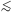3H010-42 GeV, even though the field amplitude is typically of order the Planck scale,~ 1019 GeV. This hierarchy, m/~ 10-60, means that the scalar field potential must be extremely flat. Moreover, in order not to spoil this flatness, the interaction strength of the field with itself must be extremely weak, at most of order 10-120 in dimensionless units; its coupling to matter must also be very weak to be consistent with constraints upon new long-range forces [Carroll 1998]. Understanding such very small numbers and ratios makes it challenging to connect scalar field dark energy with particle physics models [Frieman et al. 1995]. In constructing theories that go beyond the standard model of particle physics, including those that incorporate primordial inflation, model-builders have been strongly guided by the requirement that any small dimensionless numbers in the theory should be protected by symmetries from large quantum corrections (as in the SUSY example above). Thus far, such model-building discipline has not been the rule among cosmologists working on dark energy models.

A very different approach holds that cosmic acceleration is a manifestation of new gravitational physics rather than dark energy, i.e., that it involves a modification of the geometric as opposed to the stress-tensor side of the Einstein equations. Assuming that 4-d spacetime can still be described by a metric, the operational changes are twofold: (1) a new version of the Friedmann equation governing the evolution of a(t); (2) modifications to the equations that govern the growth of the density perturbations that evolve into large-scale structure. A number of ideas have been explored along these lines, from models motivated by higher-dimensional theories and string theory [Dvali, Gabadadze & Porrati 2000, Deffayet 2001] to phenomenological modifications of the Einstein-Hilbert Lagrangian of GR [Carroll et al. 2004, Song, Hu & Sawicki 2007].

Changes to the Friedmann equation are easier to derive, discuss, and analyze. In order not to spoil the success of the standard cosmology at early times (from big bang nucleosynthesis to the CMB anisotropy to the formation of structure), the Friedmann equation must reduce to the GR form for z ≫ 1. As a specific example, consider the model of [Dvali, Gabadadze & Porrati2000], which arises from a five-dimensional gravity theory and has a 4-d Friedmann equation,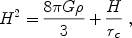(21)

where rc is a length scale related to the 5-dimensional gravitational constant. As the energy density in matter and radiation,, becomes small, there is an accelerating solution, with H = 1 / rc. From the viewpoint of expansion, the additional term in the Friedmann equation has the same effect as dark energy that has an equation-of-state parameter which evolves from w = -1/2 (for z ≫ 1) to w = -1 in the distant future. While attractive, it is not clear that a consistent model with this dynamical behavior exists (e.g., [Gregory et al. 2007]).

Instead of modifying the right or left side of the Einstein equations to explain the supernova observations, a third logical possibility is to drop the assumption that the Universe is spatially homogeneous on large scales. It has been argued that the non-linear gravitational effects of spatial density perturbations, when averaged over large scales, could yield a distance-redshift relation in our observable patch of the Universe that is very similar to that for an accelerating, homogeneous Universe [Kolb, Matarrese & Riotto 2006], obviating the need for either dark energy or modified gravity. While there has been debate about the amplitude of these effects, this idea has helped spark renewed interest in a class of exact, inhomogeneous cosmologies. For such Lemaître-Tolman-Bondi models to be consistent with the SN data and not conflict with the isotropy of the CMB, the Milky Way must be near the center of a very large-scale, nearly spherical, underdense region [Tomita 2001, Alnes, Amarzguioui & Gron 2006, Enqvist 2007]. Whether or not such models can be made consistent with the wealth of precision cosmological data remains to be seen; moreover, requiring our galaxy to occupy a privileged location, in violation of the spirit of the Copernican principle, is not yet theoretically well-motivated.

There is no compelling explanation for cosmic acceleration, but many intriguing ideas are being explored. Here is our assessment:

• Cosmological constant: Simple, but no underlying physics
• Vacuum energy: Well-motivated, mathematically equivalent to a cosmological constant; w = -1 is consistent with all data, but all attempts to estimate its size are at best orders of magnitude too large
• Scalar fields: Temporary period of cosmic acceleration, w varies between -1 and 1 (and could also be < -1), possibly related to inflation, but does not address the cosmological constant problem and may lead to new long-range forces
• New gravitational physics: Cosmic acceleration could be a clue to going beyond GR, but no self-consistent model has been put forth
• Old gravitational physics: It may be possible to find an inhomogeneous solution that is observationally viable, but such solutions do not yet seem compelling

The ideas underlying many of these approaches, from attempting to explain the smallness of quantum vacuum energy to extending Einstein's theory, are bold. Solving the puzzle of cosmic acceleration thus has the potential to advance our understanding of many important problems in fundamental physics.